# R S Aggarwal Solutions for Class 10 Maths Chapter 4 Quadratic Equations Exercise 4A

R S Aggarwal Solutions for Class 10 Maths Chapter 4 Quadratic Equations exercise 4A are provided here. This exercise helps students in solving quadratic equations by factorisation. Subject experts have crafted all the solutions using images and graphs for clear understanding. Download free R S Aggarwal Solutions of Class 10 pdf for Maths and score well in the exams.

## Download PDF of R S Aggarwal Solutions for Class 10 Maths Chapter 4 Quadratic Equations Exercise 4A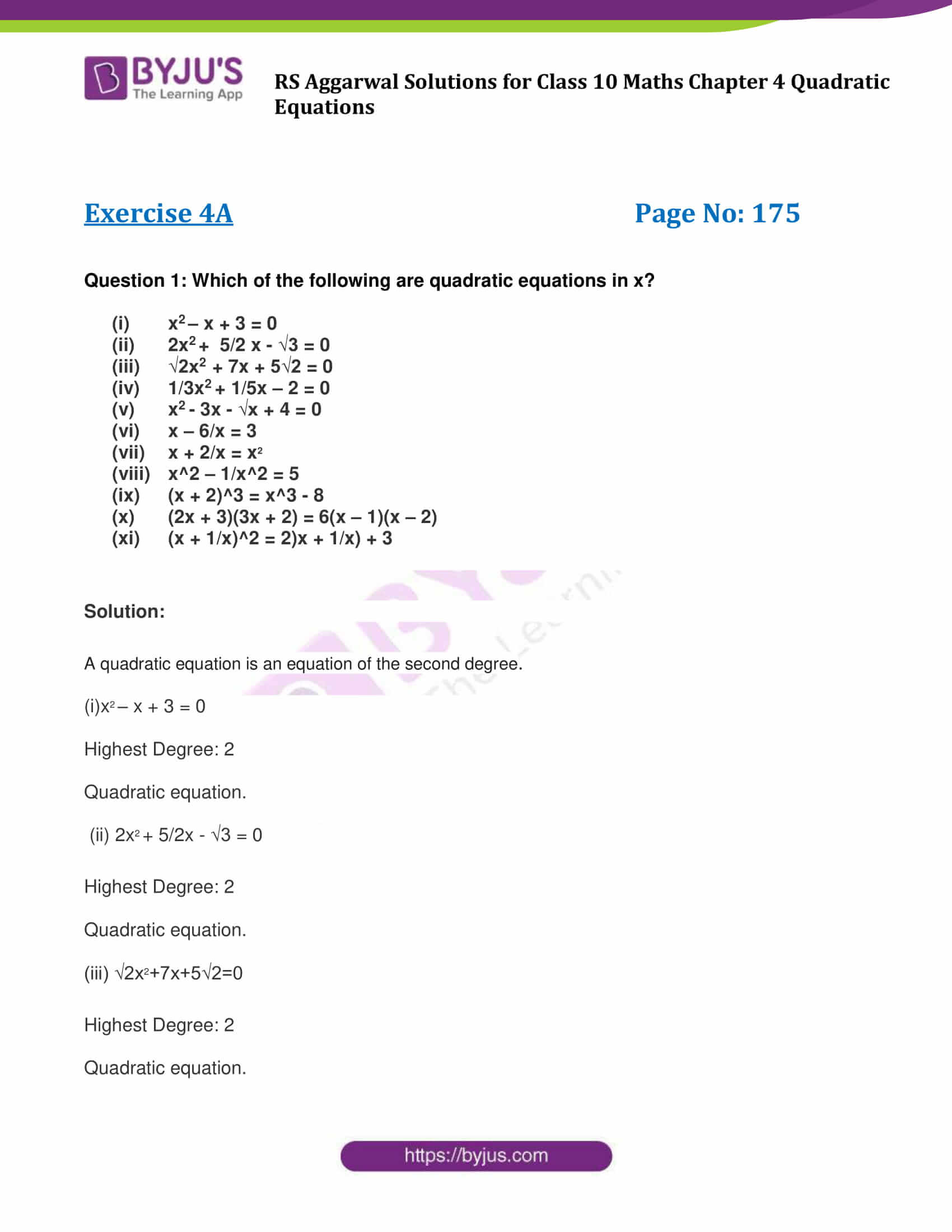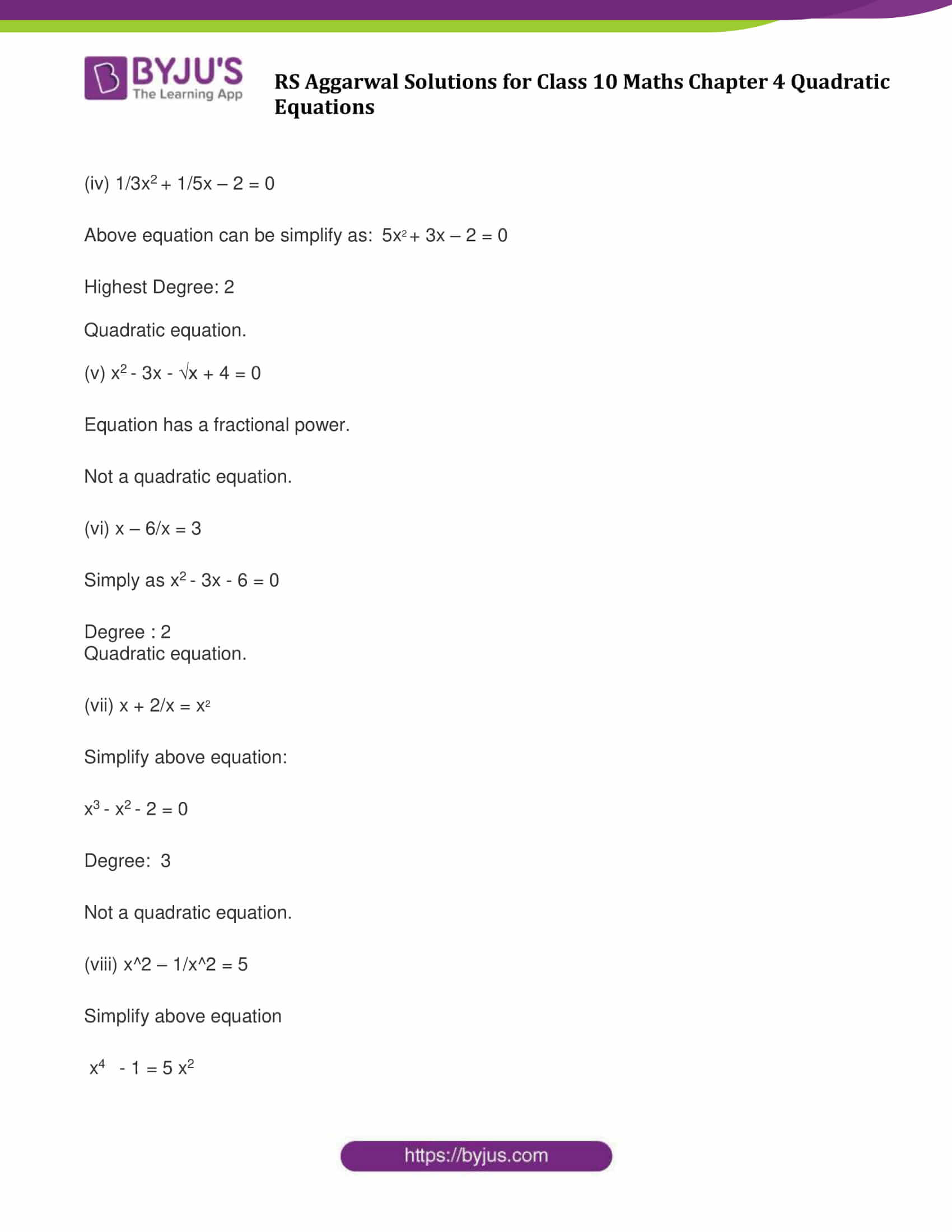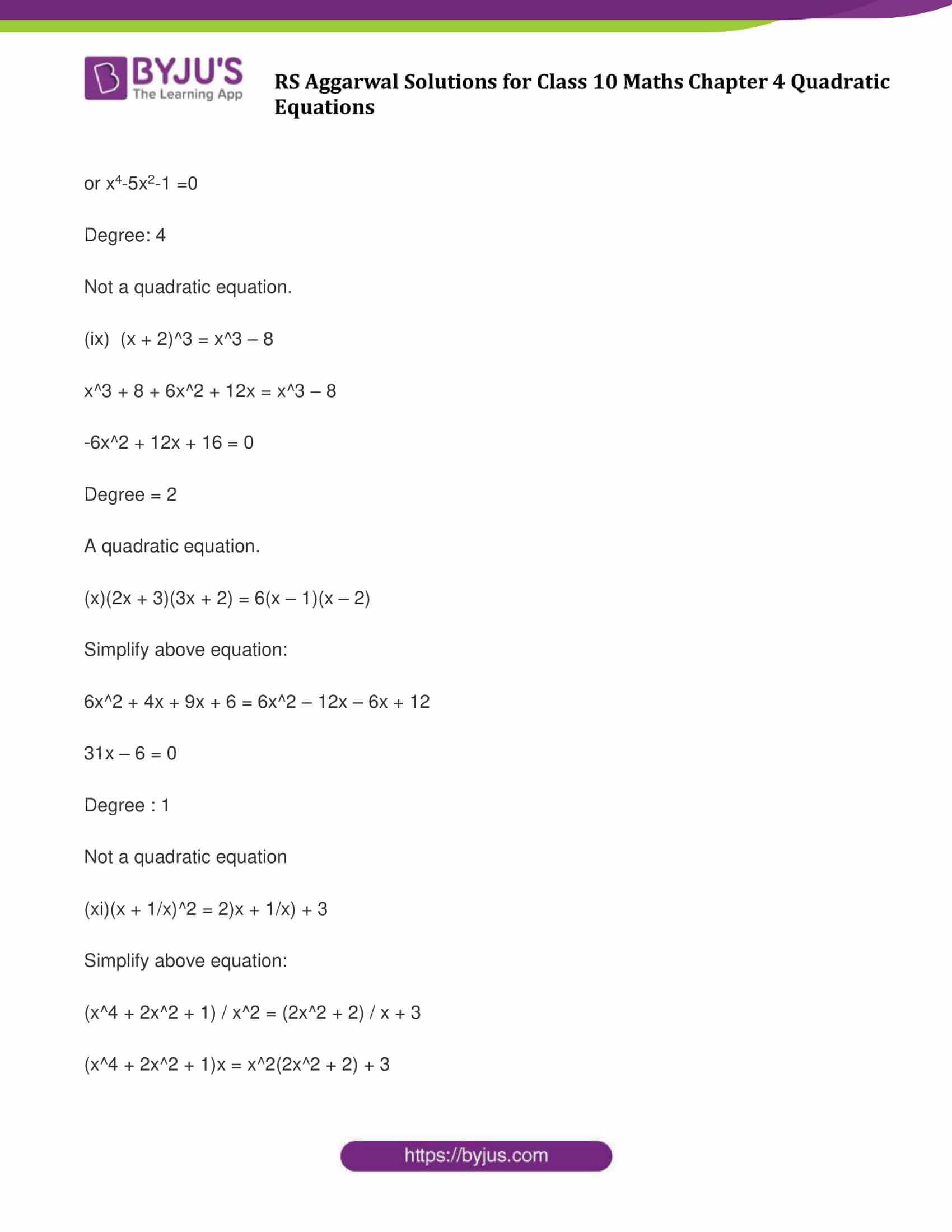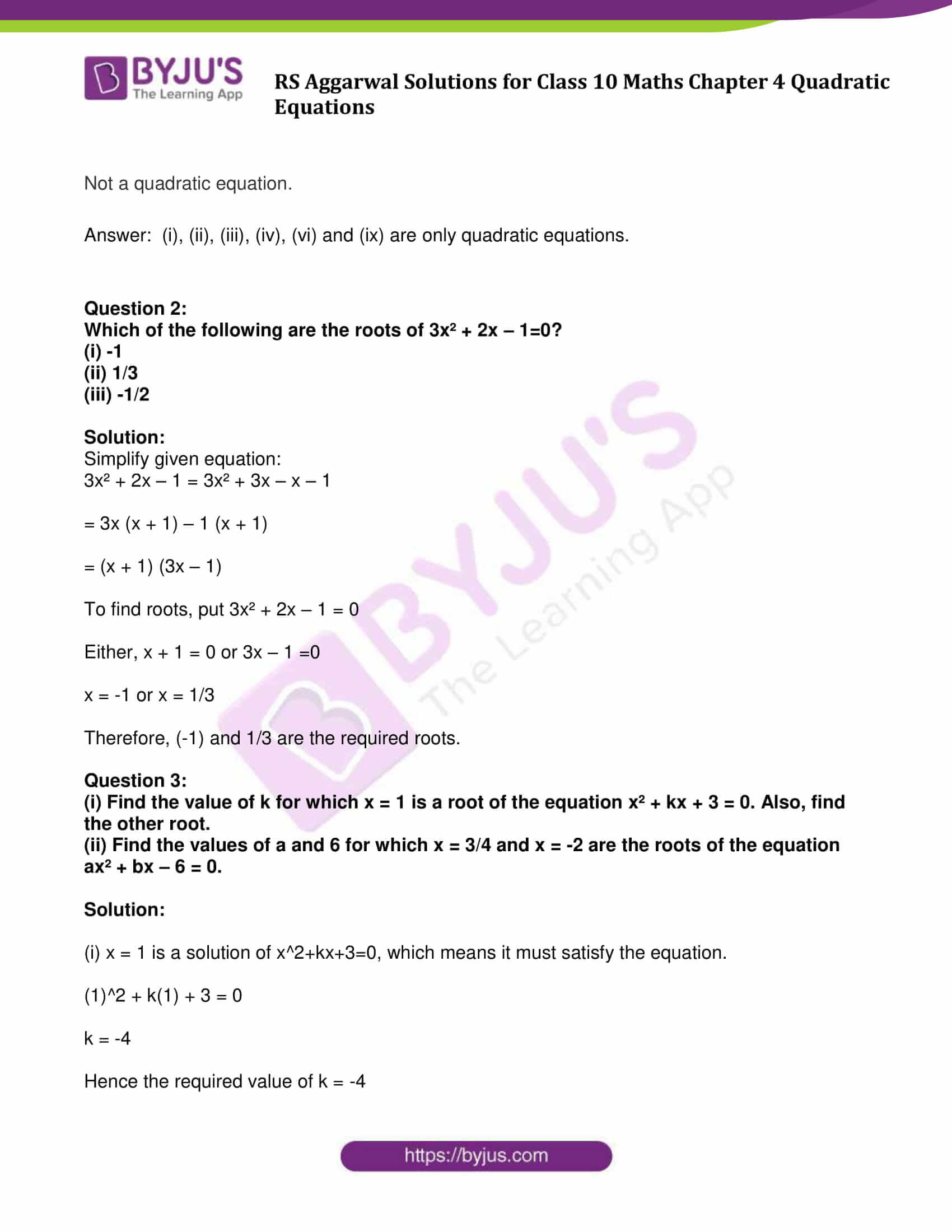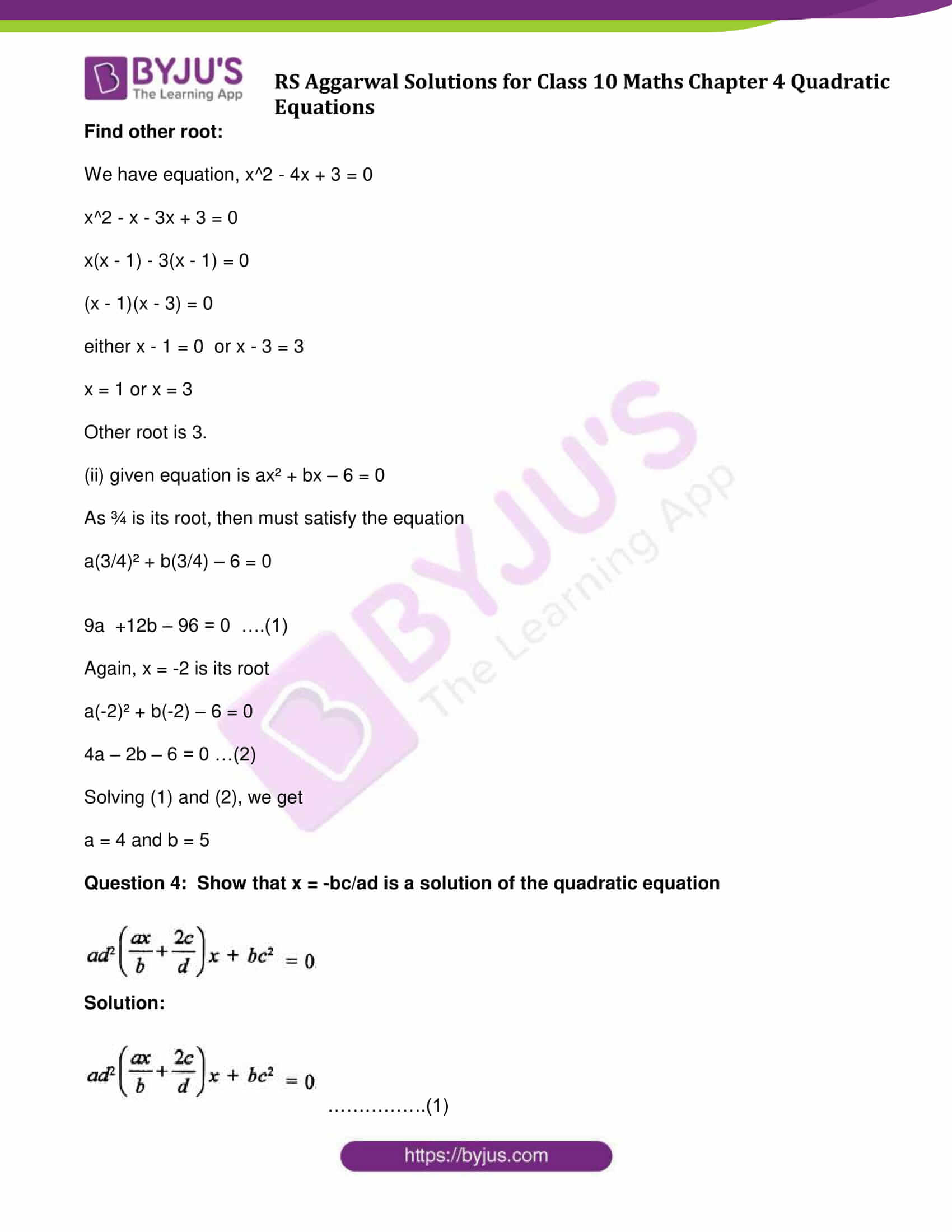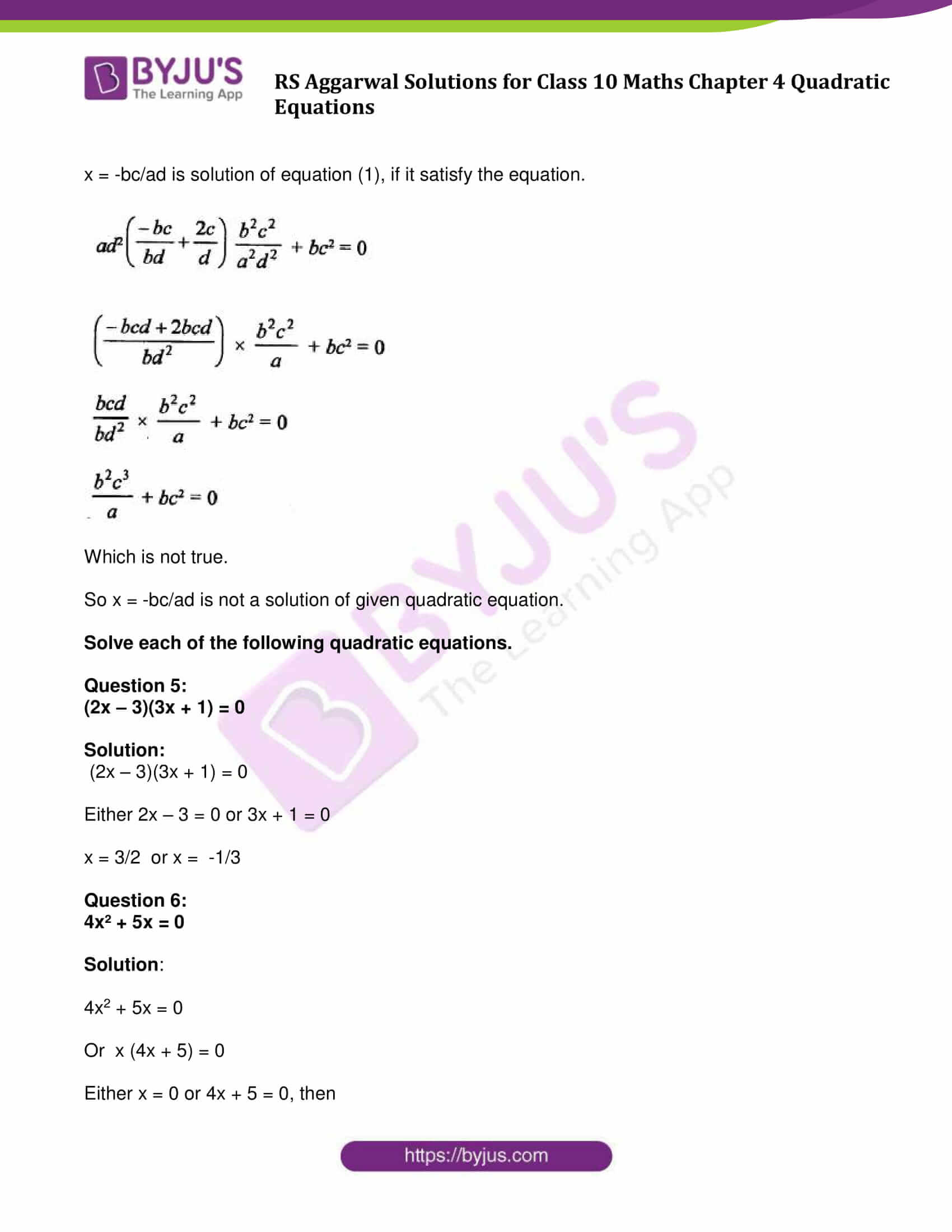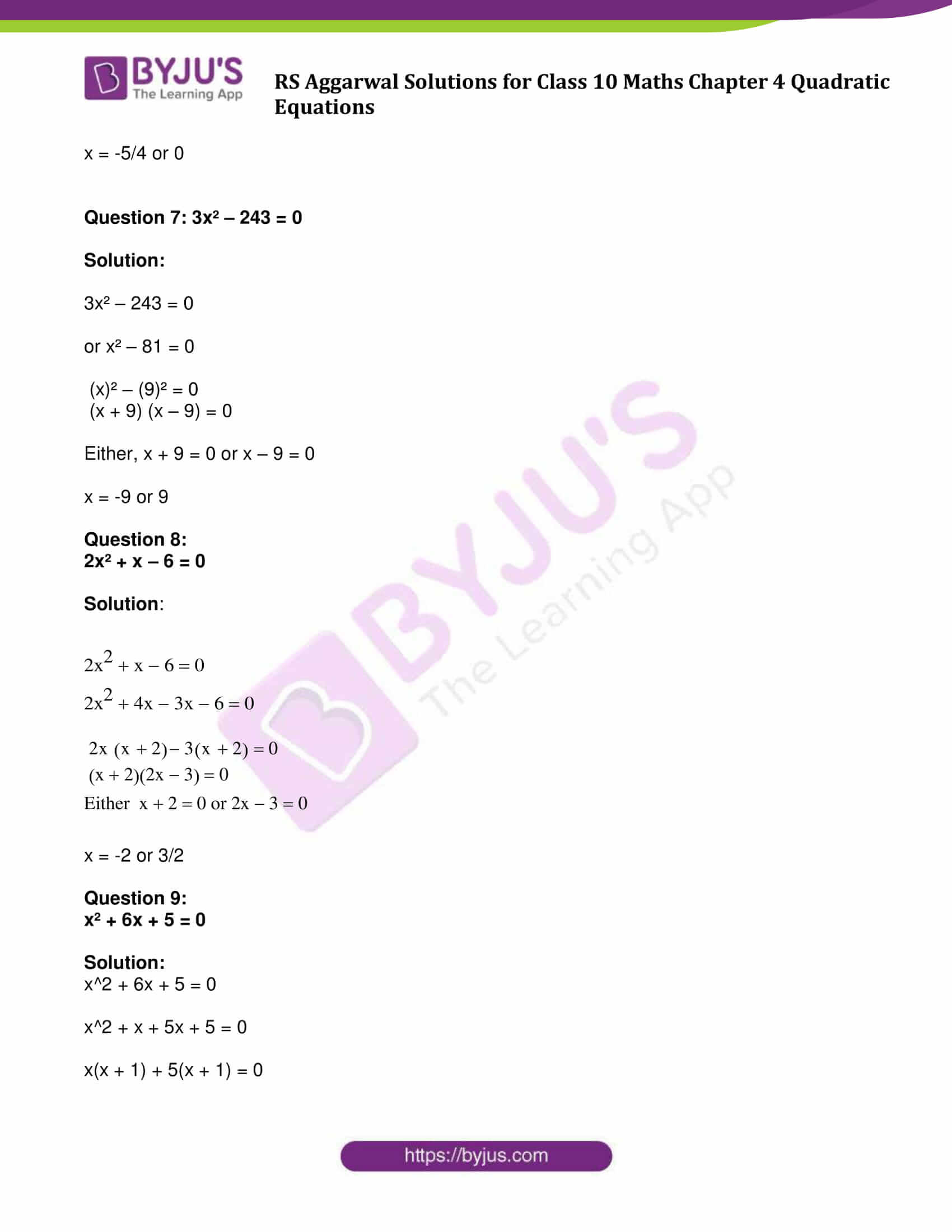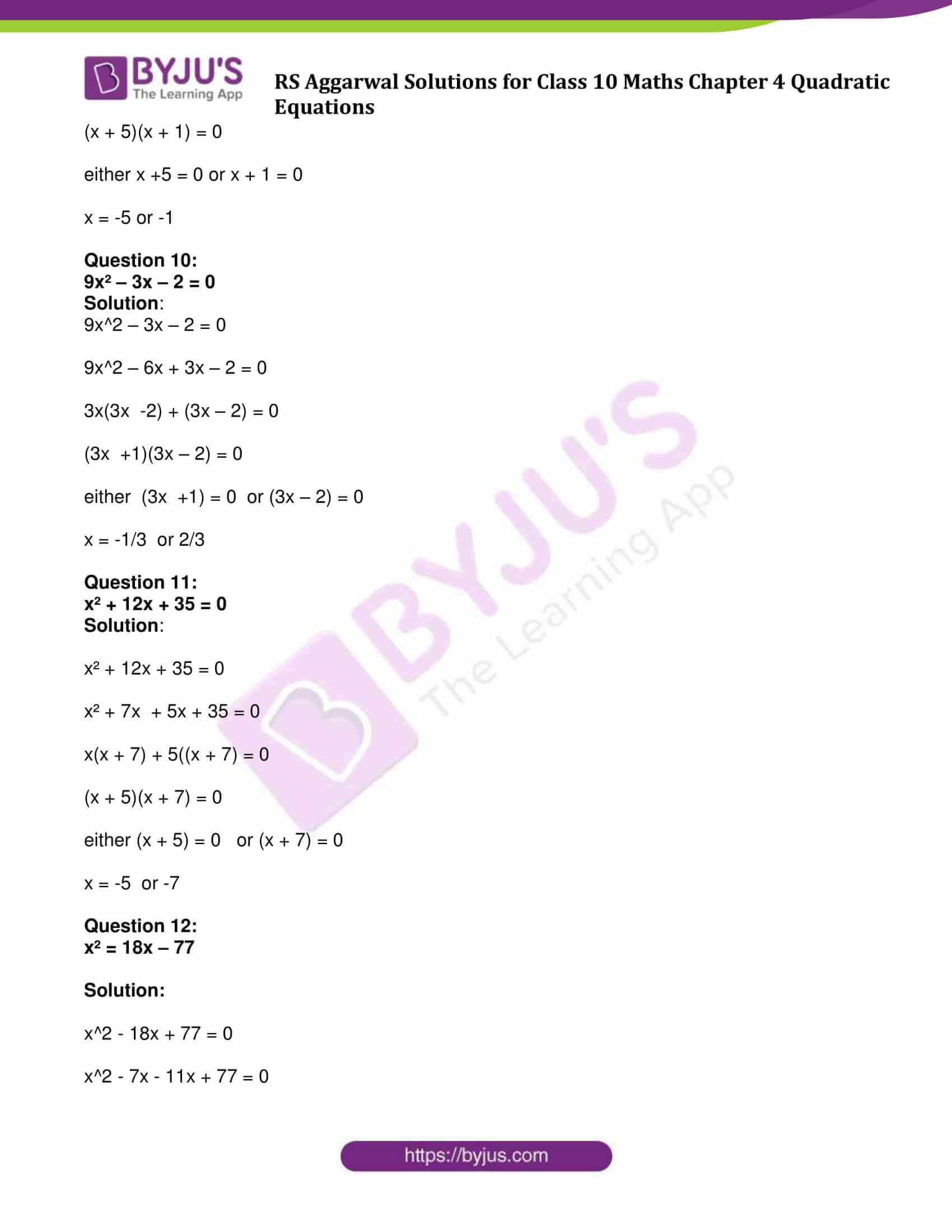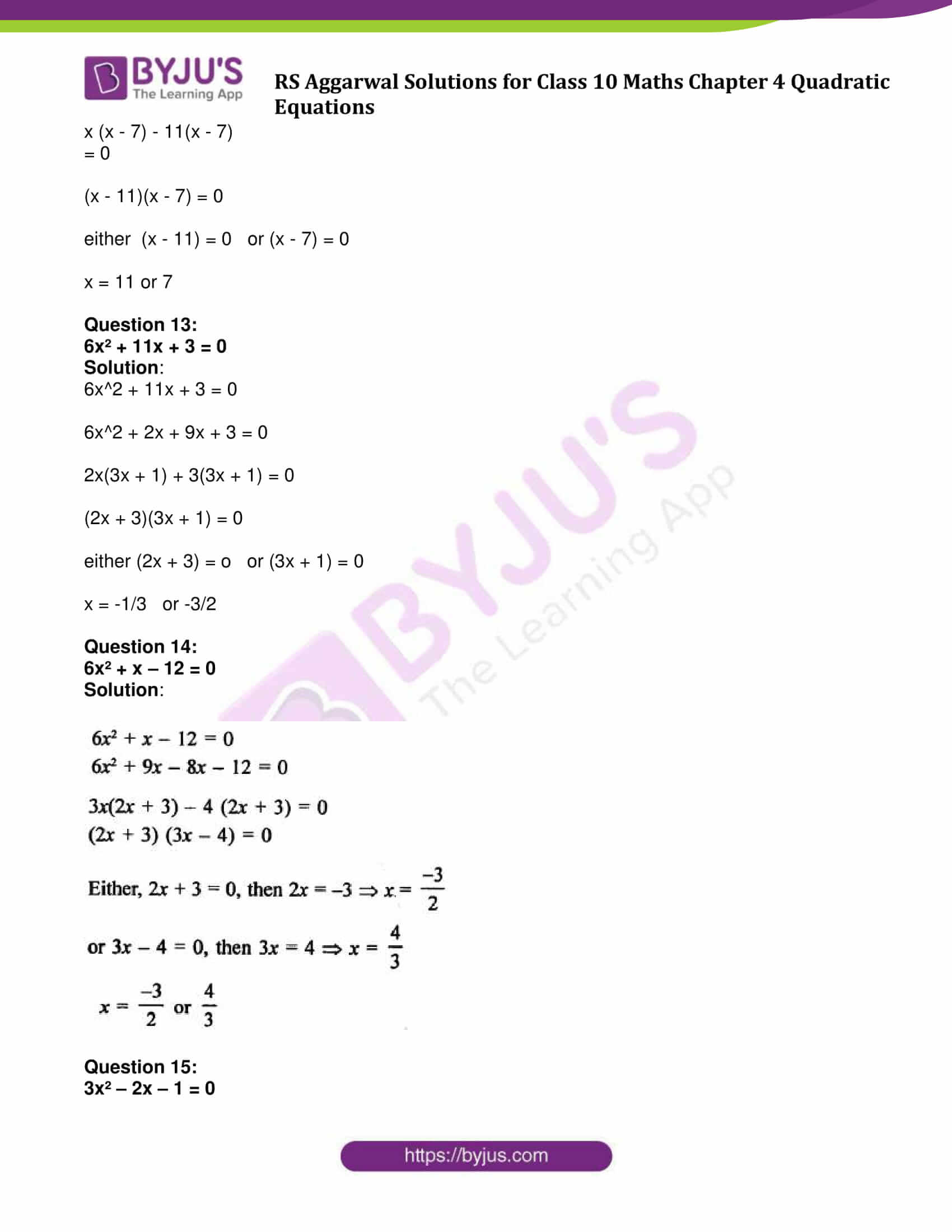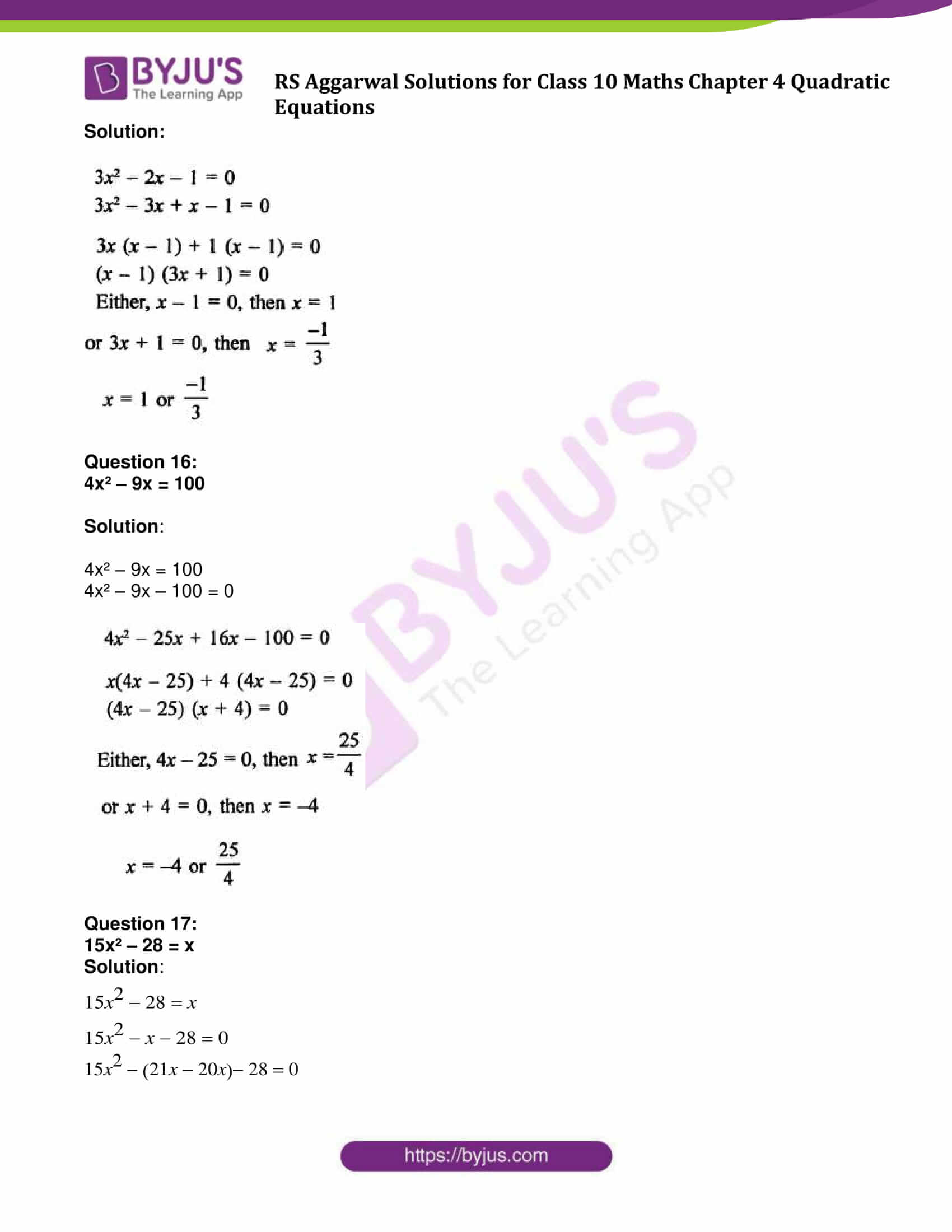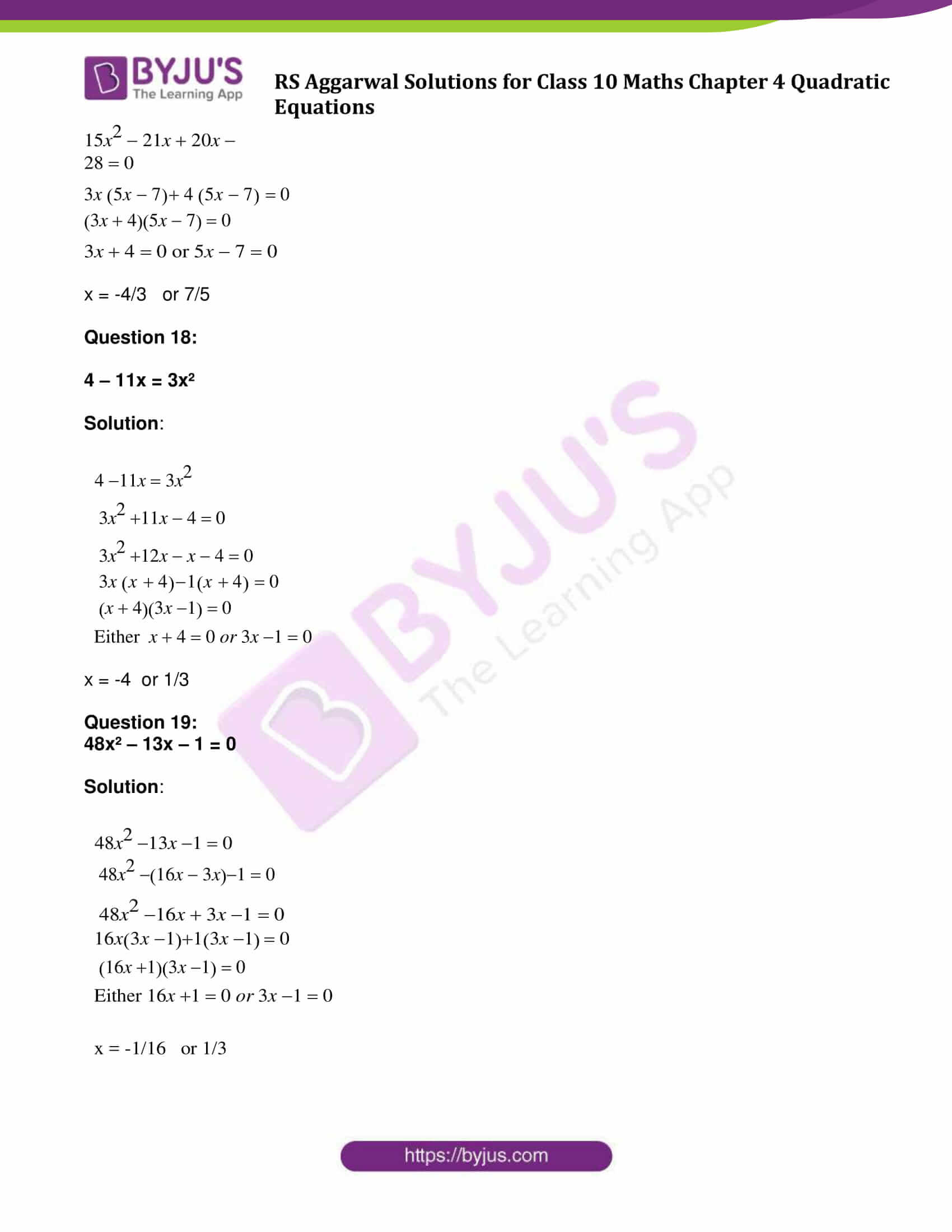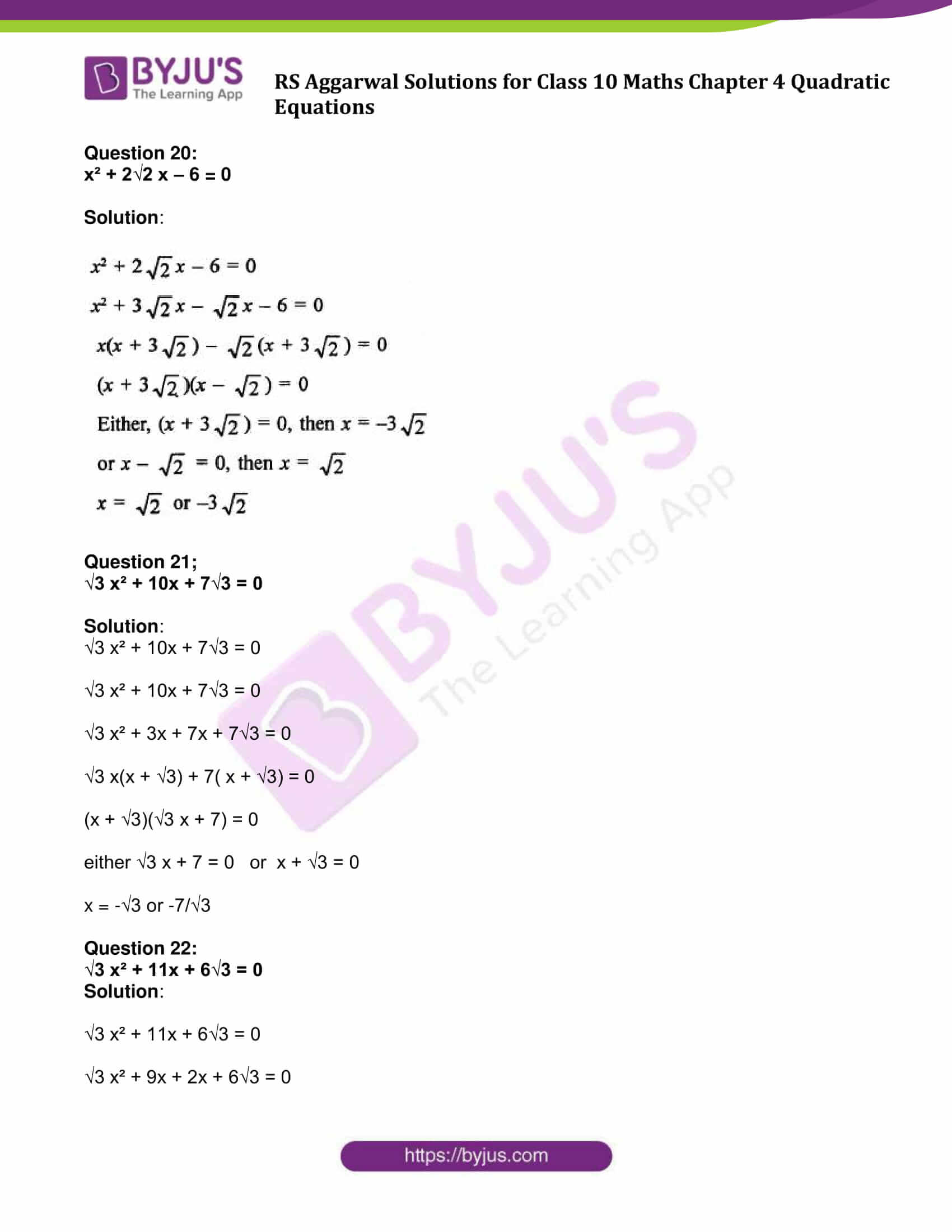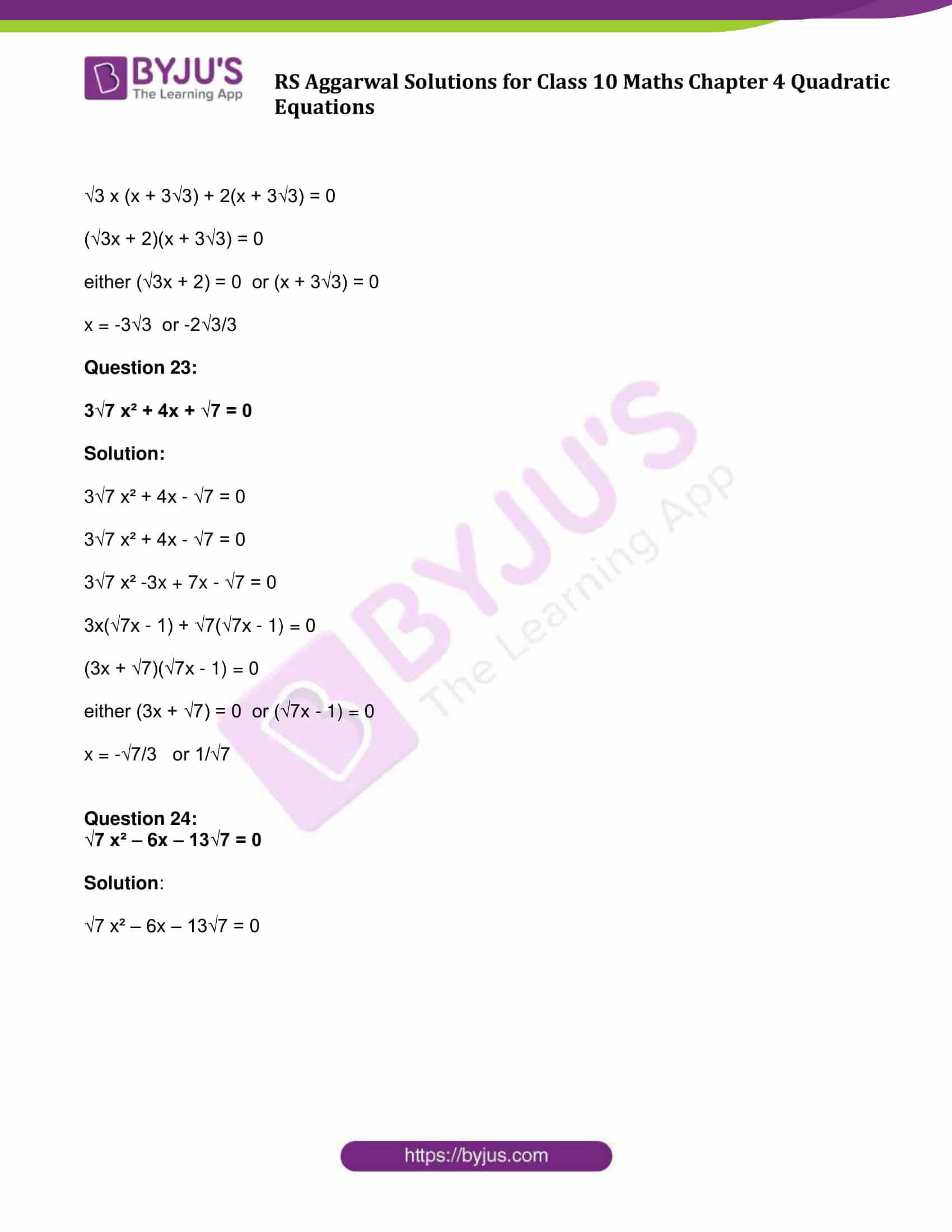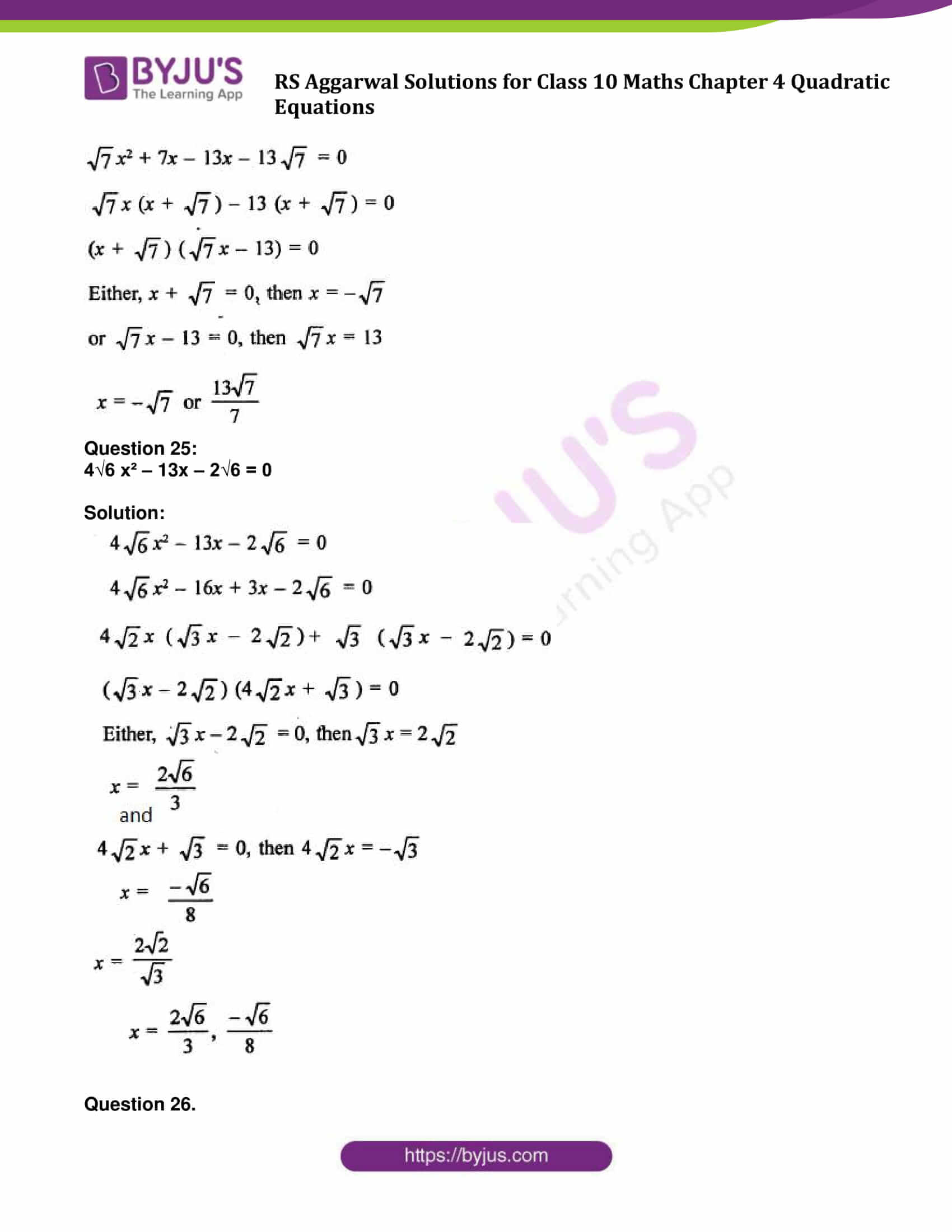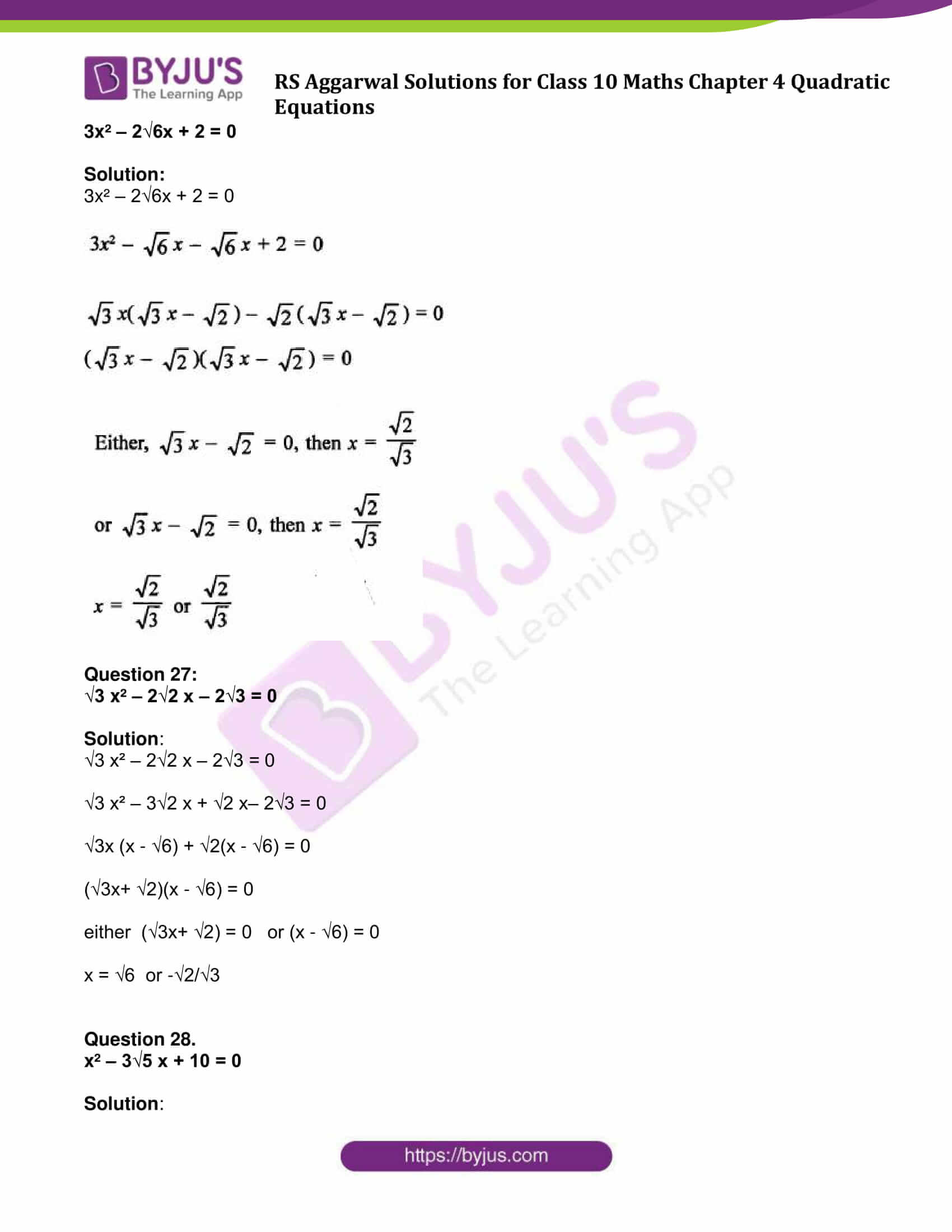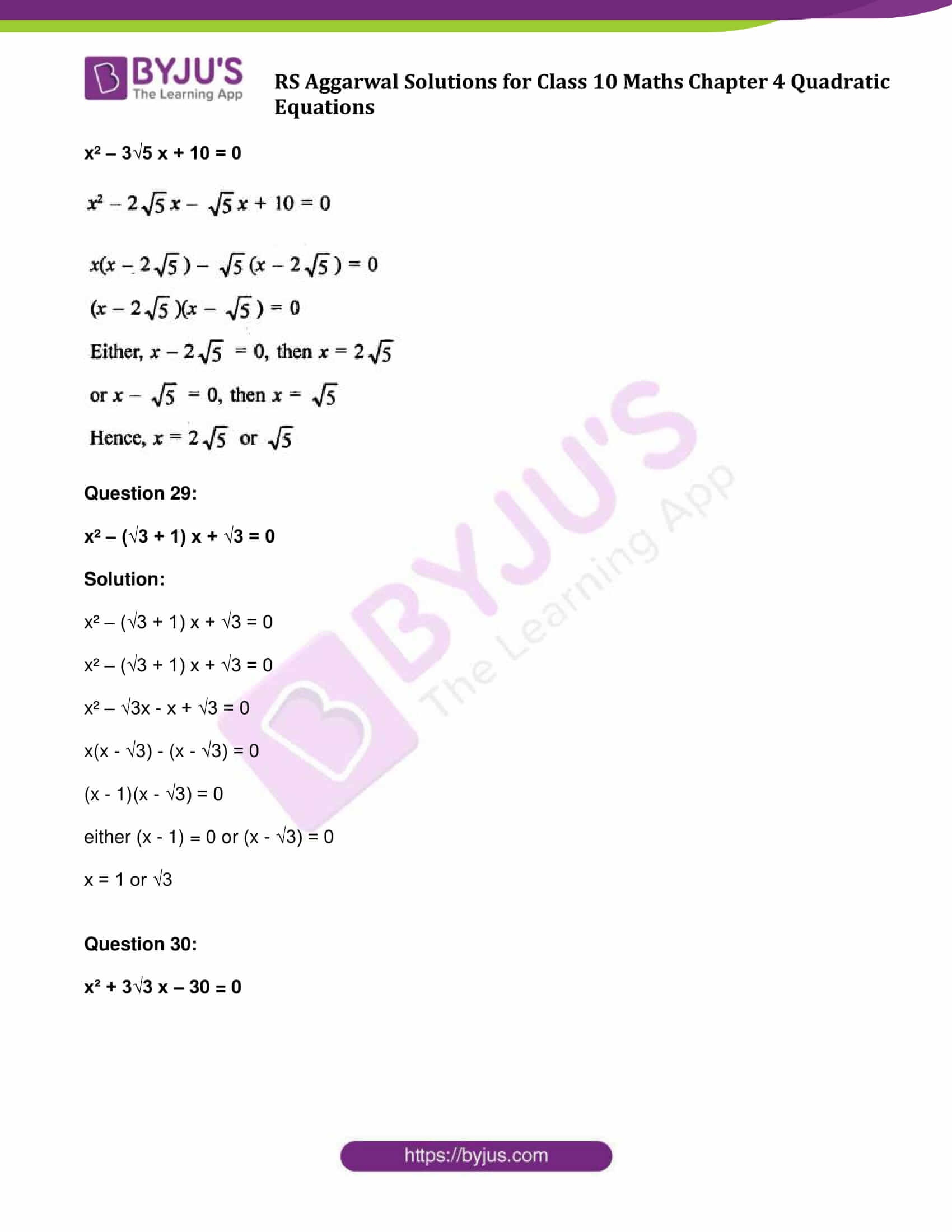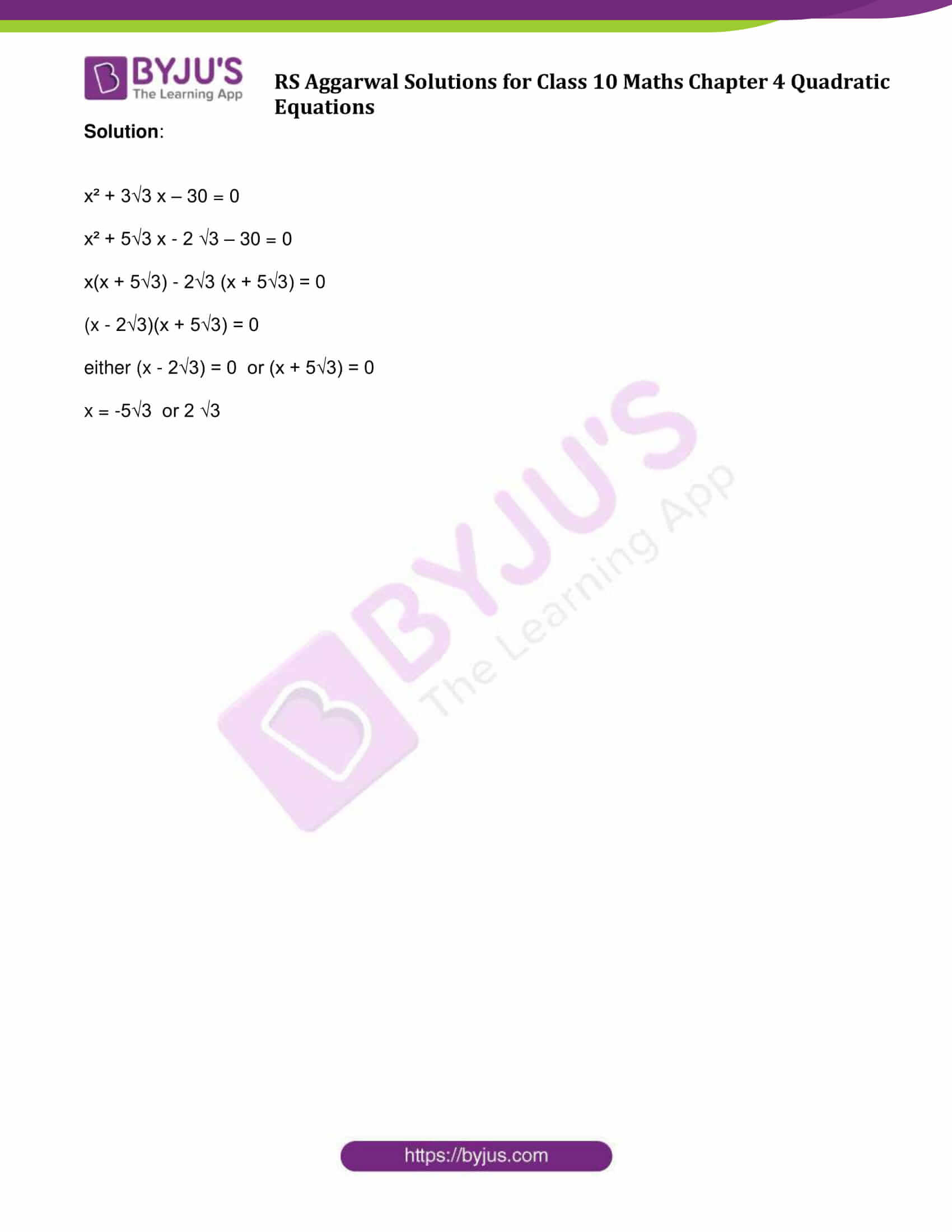### Access other exercise solutions of Class 10 Maths Chapter 4 Quadratic Equations

Exercise 4B Solutions: 16 Questions (Short Answers)

Exercise 4C Solutions: 15 Questions (Short Answers)

Exercise 4D Solutions: 20 Questions (Long Answers)

## Exercise 4A Page No: 175

Question 1: Which of the following are quadratic equations in x?

1. x2 – x + 3 = 0
2. 2x2 +  5/2 x – √3 = 0
3. √2x2 + 7x + 5√2 = 0
4. 1/3x2 + 1/5x – 2 = 0
5. x2 – 3x – √x + 4 = 0
6. x – 6/x = 3
7. x + 2/x = x2
8. x^2 – 1/x^2 = 5
9. (x + 2)^3 = x^3 – 8
10. (2x + 3)(3x + 2) = 6(x – 1)(x – 2)
11. (x + 1/x)^2 = 2)x + 1/x) + 3

Solution:

A quadratic equation is an equation of the second degree.

(i) x2 – x + 3 = 0

Highest Degree: 2

(ii) 2x2 + 5/2x – √3 = 0

Highest Degree: 2

(iii) √2x2+7x+5√2=0

Highest Degree: 2

(iv) 1/3x2 + 1/5x – 2 = 0

Above equation can be simplify as: 5x2 + 3x – 2 = 0

Highest Degree: 2

(v) x2 – 3x – √x + 4 = 0

Equation has a fractional power.

(vi) x – 6/x = 3

Simply as x2 – 3x – 6 = 0

Degree : 2

(vii) x + 2/x = x2

Simplify above equation:

x3 – x2 – 2 = 0

Degree: 3

(viii) x^2 – 1/x^2 = 5

Simplify above equation

x4   – 1 = 5 x2

or x4-5x2-1 =0

Degree: 4

(ix) (x + 2)^3 = x^3 – 8

x^3 + 8 + 6x^2 + 12x = x^3 – 8

-6x^2 + 12x + 16 = 0

Degree = 2

(x) (2x + 3)(3x + 2) = 6(x – 1)(x – 2)

Simplify above equation:

6x^2 + 4x + 9x + 6 = 6x^2 – 12x – 6x + 12

31x – 6 = 0

Degree : 1

(xi) (x + 1/x)^2 = 2)x + 1/x) + 3

Simplify above equation:

(x^4 + 2x^2 + 1) / x^2 = (2x^2 + 2) / x + 3

(x^4 + 2x^2 + 1)x = x^2(2x^2 + 2) + 3

Answer: (i), (ii), (iii), (iv), (vi) and (ix) are only quadratic equations.

Question 2:

Which of the following are the roots of 3x² + 2x – 1=0?

(i) -1

(ii) 1/3

(iii) -1/2

Solution:

Simplify given equation:

3x² + 2x – 1 = 3x² + 3x – x – 1

= 3x (x + 1) – 1 (x + 1)

= (x + 1) (3x – 1)

To find roots, put 3x² + 2x – 1 = 0

Either, x + 1 = 0 or 3x – 1 =0

x = -1 or x = 1/3

Therefore, (-1) and 1/3 are the required roots.

Question 3:

(i) Find the value of k for which x = 1 is a root of the equation x² + kx + 3 = 0. Also, find the other root.

(ii) Find the values of a and 6 for which x = 3/4 and x = -2 are the roots of the equation ax² + bx – 6 = 0.

Solution:

(i) x = 1 is a solution of x^2+kx+3=0, which means it must satisfy the equation.

(1)^2 + k(1) + 3 = 0

k = -4

Hence the required value of k = -4

Find other root:

We have equation, x^2 – 4x + 3 = 0

x^2 – x – 3x + 3 = 0

x(x – 1) – 3(x – 1) = 0

(x – 1)(x – 3) = 0

either x – 1 = 0 or x – 3 = 3

x = 1 or x = 3

Other root is 3.

(ii) given equation is ax² + bx – 6 = 0

As ¾ is its root, then must satisfy the equation

a(3/4)² + b(3/4) – 6 = 0

9a +12b – 96 = 0 ….(1)

Again, x = -2 is its root

a(-2)² + b(-2) – 6 = 0

4a – 2b – 6 = 0 …(2)

Solving (1) and (2), we get

a = 4 and b = 5

Question 4: Show that x = -bc/ad is a solution of the quadratic equation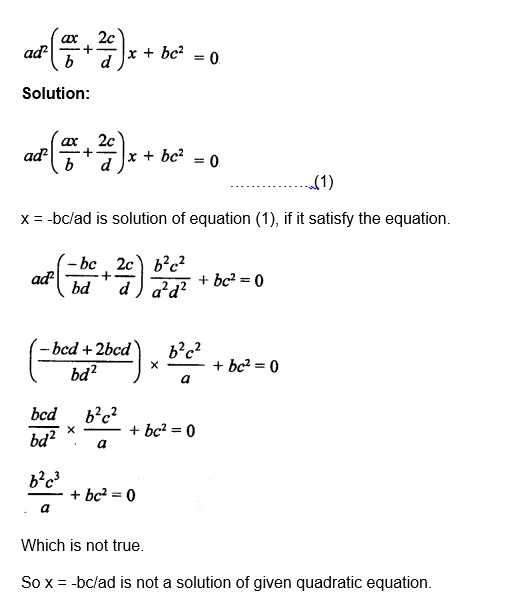Solve each of the following quadratic equations.

Question 5:

(2x – 3)(3x + 1) = 0

Solution:

(2x – 3)(3x + 1) = 0

Either 2x – 3 = 0 or 3x + 1 = 0

x = 3/2 or x = -1/3

Question 6: 4x² + 5x = 0

Solution:

4x2 + 5x = 0

Or x (4x + 5) = 0

Either x = 0 or 4x + 5 = 0, then

x = -5/4 or 0

Question 7: 3x² – 243 = 0

Solution:

3x² – 243 = 0

or x² – 81 = 0

(x)² – (9)² = 0

(x + 9) (x – 9) = 0

Either, x + 9 = 0 or x – 9 = 0

x = -9 or 9

Question 8: 2x² + x – 6 = 0

Solution:

2×2  x  6  0

2×2  4x  3x  6  0

2x x  2  3x  2  0

x  22x  3  0

Either x  2  0 or 2x  3  0

x = -2 or 3/2

Question 9: x² + 6x + 5 = 0

Solution:

x^2 + 6x + 5 = 0

x^2 + x + 5x + 5 = 0

x(x + 1) + 5(x + 1) = 0

(x + 5)(x + 1) = 0

either x +5 = 0 or x + 1 = 0

x = -5 or -1

Question 10: 9x² – 3x – 2 = 0

Solution:

9x^2 – 3x – 2 = 0

9x^2 – 6x + 3x – 2 = 0

3x(3x -2) + (3x – 2) = 0

(3x +1)(3x – 2) = 0

either (3x +1) = 0 or (3x – 2) = 0

x = -1/3 or 2/3

Question 11: x² + 12x + 35 = 0

Solution:

x² + 12x + 35 = 0

x² + 7x + 5x + 35 = 0

x(x + 7) + 5((x + 7) = 0

(x + 5)(x + 7) = 0

either (x + 5) = 0 or (x + 7) = 0

x = -5 or -7

Question 12: x² = 18x – 77

Solution:

x^2 – 18x + 77 = 0

x^2 – 7x – 11x + 77 = 0

x (x – 7) – 11(x – 7) = 0

(x – 11)(x – 7) = 0

either (x – 11) = 0 or (x – 7) = 0

x = 11 or 7

Question 13: 6x² + 11x + 3 = 0

Solution:

6x^2 + 11x + 3 = 0

6x^2 + 2x + 9x + 3 = 0

2x(3x + 1) + 3(3x + 1) = 0

(2x + 3)(3x + 1) = 0

either (2x + 3) = o or (3x + 1) = 0

x = -1/3 or -3/2

Question 14: 6x² + x – 12 = 0

Solution: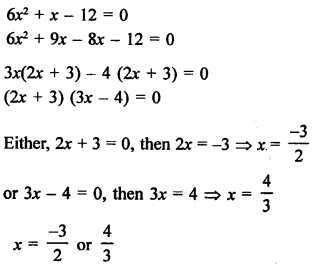Question 15:

3x² – 2x – 1 = 0

Solution: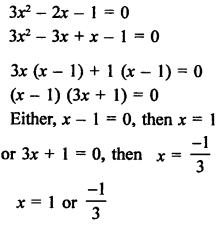Question 16:

4x² – 9x = 100

Solution:

4x² – 9x = 100

4x² – 9x – 100 = 0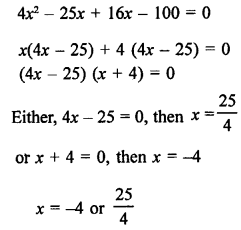Question 17:

15x² – 28 = x

Solution:

15x2  28  x

15x2  x  28  0

15x2  21x  20x 28  0

15x2  21x  20x  28  0

3x 5x  7  4 5x  7  0

3x  45x  7  0

3x  4  0 or 5x  7  0

x = -4/3 or 7/5

Question 18:

4 – 11x = 3x²

Solution:

4 11x  3x2

3x2 11x  4  0

3x2 12x x  4  0

3x x  4 1x  4  0

x  43x 1  0

Either x  4  0 or 3x 1  0

x = -4 or 1/3

Question 19: 48x² – 13x – 1 = 0

Solution:

48x2 13x 1  0

48x2 16x  3x1  0

48x2 16x  3x 1  0

16x3x 113x 1  0

16x 13x 1  0

Either 16x 1  0 or 3x 1  0

x = -1/16 or 1/3

Question 20:

x² + 2√2 x – 6 = 0

Solution: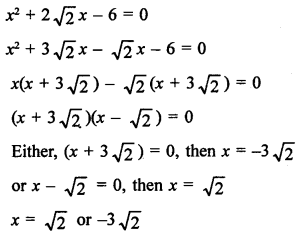Question 21;

√3 x² + 10x + 7√3 = 0

Solution:

√3 x² + 10x + 7√3 = 0

√3 x² + 10x + 7√3 = 0

√3 x² + 3x + 7x + 7√3 = 0

√3 x(x + √3) + 7( x + √3) = 0

(x + √3)(√3 x + 7) = 0

either √3 x + 7 = 0 or x + √3 = 0

x = -√3 or -7/√3

Question 22:

√3 x² + 11x + 6√3 = 0

Solution:

√3 x² + 11x + 6√3 = 0

√3 x² + 9x + 2x + 6√3 = 0

√3 x (x + 3√3) + 2(x + 3√3) = 0

(√3x + 2)(x + 3√3) = 0

either (√3x + 2) = 0 or (x + 3√3) = 0

x = -3√3 or -2√3/3

Question 23:

3√7 x² + 4x + √7 = 0

Solution:

3√7 x² + 4x – √7 = 0

3√7 x² + 4x – √7 = 0

3√7 x² -3x + 7x – √7 = 0

3x(√7x – 1) + √7(√7x – 1) = 0

(3x + √7)(√7x – 1) = 0

either (3x + √7) = 0 or (√7x – 1) = 0

x = -√7/3 or 1/√7

Question 24:

√7 x² – 6x – 13√7 = 0

Solution:

√7 x² – 6x – 13√7 = 0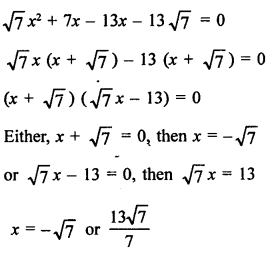Question 25: 4√6 x² – 13x – 2√6 = 0

Solution: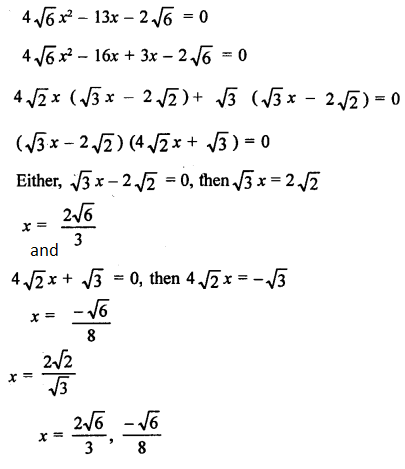Question 26: 3x² – 2√6x + 2 = 0

Solution:

3x² – 2√6x + 2 = 0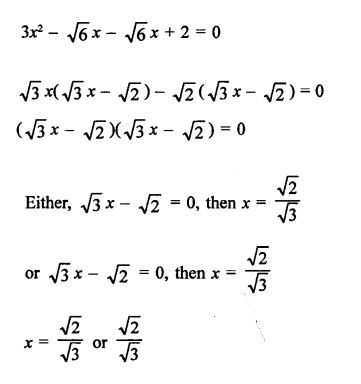Question 27: √3 x² – 2√2 x – 2√3 = 0

Solution:

√3 x² – 2√2 x – 2√3 = 0

√3 x² – 3√2 x + √2 x– 2√3 = 0

√3x (x – √6) + √2(x – √6) = 0

(√3x+ √2)(x – √6) = 0

either (√3x+ √2) = 0 or (x – √6) = 0

x = √6 or -√2/√3

Question 28: x² – 3√5 x + 10 = 0

Solution: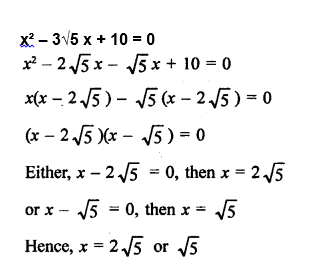Question 29:

x² – (√3 + 1) x + √3 = 0

Solution:

x² – (√3 + 1) x + √3 = 0

x² – (√3 + 1) x + √3 = 0

x² – √3x – x + √3 = 0

x(x – √3) – (x – √3) = 0

(x – 1)(x – √3) = 0

either (x – 1) = 0 or (x – √3) = 0

x = 1 or √3

Question 30:

x² + 3√3 x – 30 = 0

Solution:

x² + 3√3 x – 30 = 0

x² + 5√3 x – 2 √3 – 30 = 0

x(x + 5√3) – 2√3 (x + 5√3) = 0

(x – 2√3)(x + 5√3) = 0

either (x – 2√3) = 0 or (x + 5√3) = 0

x = -5√3 or 2 √3

## R S Aggarwal Solutions for Class 10 Maths Chapter 4 Quadratic Equations Exercise 4A

Class 10 Maths Chapter 4 Quadratic Equations Exercise 4A is based on the topics: